This is one of the 100+ free recipes of the IPython Cookbook, Second Edition, by Cyrille Rossant, a guide to numerical computing and data science in the Jupyter Notebook. The ebook and printed book are available for purchase at Packt Publishing.

▶  Text on GitHub with a CC-BY-NC-ND license
▶  Code on GitHub with a MIT license

seaborn is a library that builds on top of matplotlib and pandas to provide easy-to-use statistical plotting routines. In this recipe, we give a few examples of the types of statistical plots that can be created with seaborn.

## How to do it...

1.  Let's import NumPy, matplotlib, and seaborn:

```import numpy as np
from scipy import stats
import matplotlib.pyplot as plt
import seaborn as sns
%matplotlib inline
```

2.  seaborn comes with builtin datasets, which are useful when making demos. The `tips` datasets contains the bills and tips of taxi trips:

```tips = sns.load_dataset('tips')
tips
```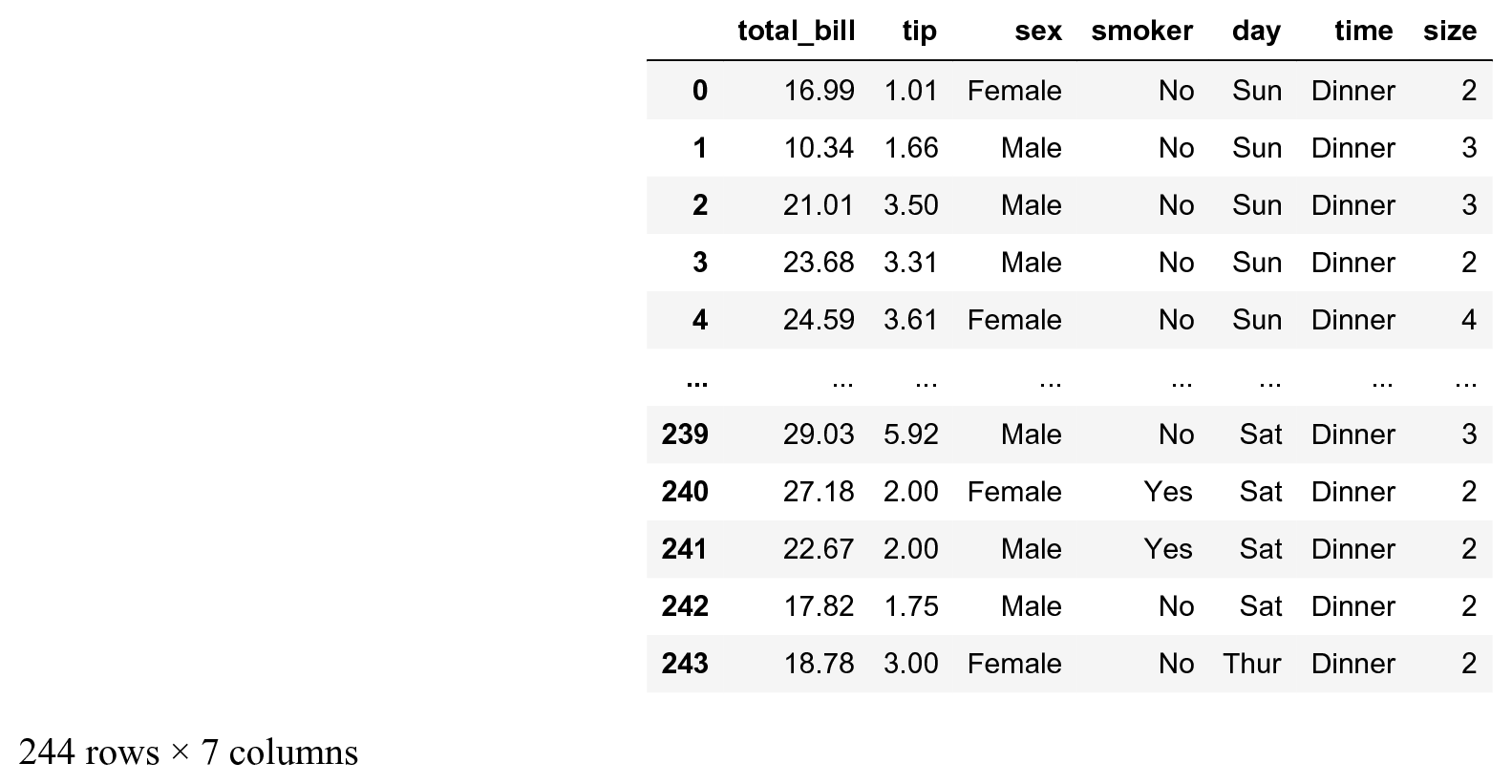3.  seaborn implements easy-to-use functions to visualize the distribution of datasets. Here, we plot the histogram, kernel density estimation (KDE), and a gamma distribution fit of our dataset:

```# We create two subplots sharing the same y axis.
f, (ax1, ax2) = plt.subplots(1, 2,
figsize=(12, 5),
sharey=True)

# Left subplot.
# Histogram and KDE (active by default).
sns.distplot(tips.total_bill,
ax=ax1,
hist=True)

# Right subplot.
# "Rugplot", KDE, and gamma fit.
sns.distplot(tips.total_bill,
ax=ax2,
hist=False,
kde=True,
rug=True,
fit=stats.gamma,
fit_kws=dict(label='gamma'),
kde_kws=dict(label='kde'))
ax2.legend()
```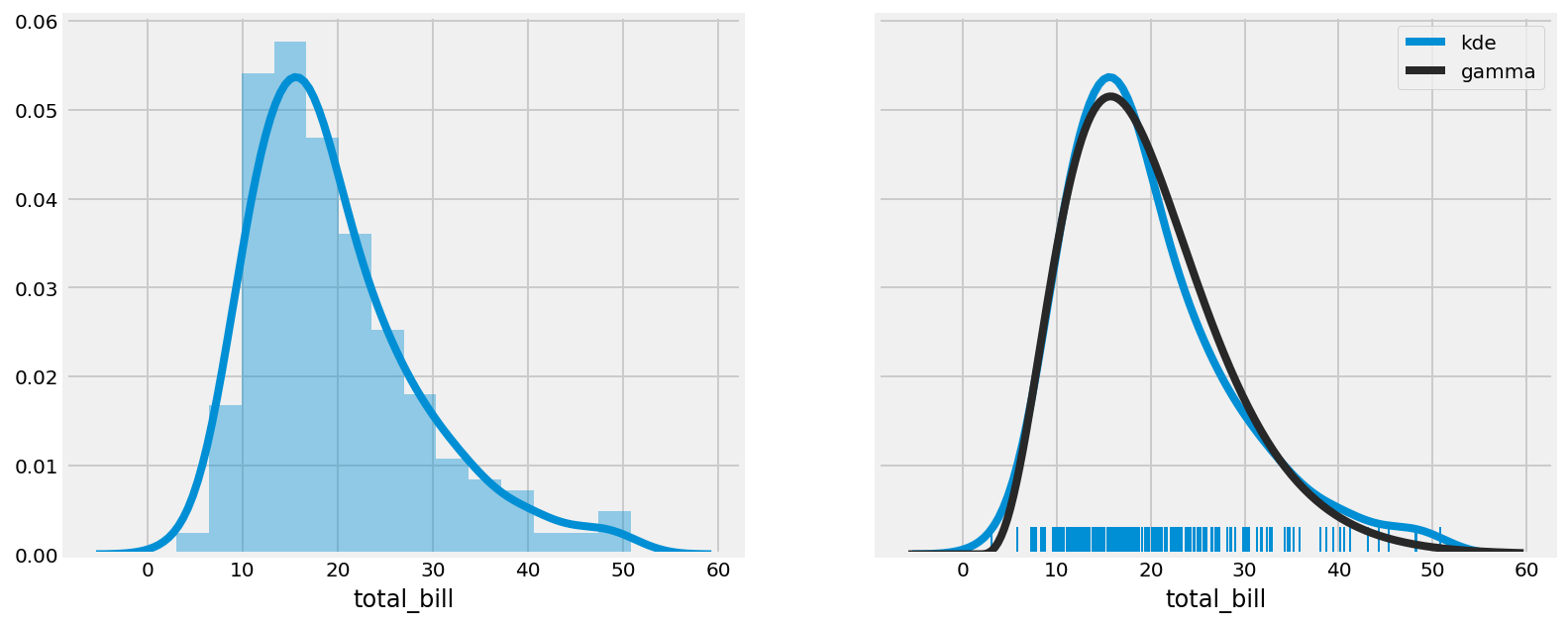4.  We can make a quick linear regression to visualize the correlation between two variables:

```sns.regplot(x="total_bill", y="tip", data=tips)
```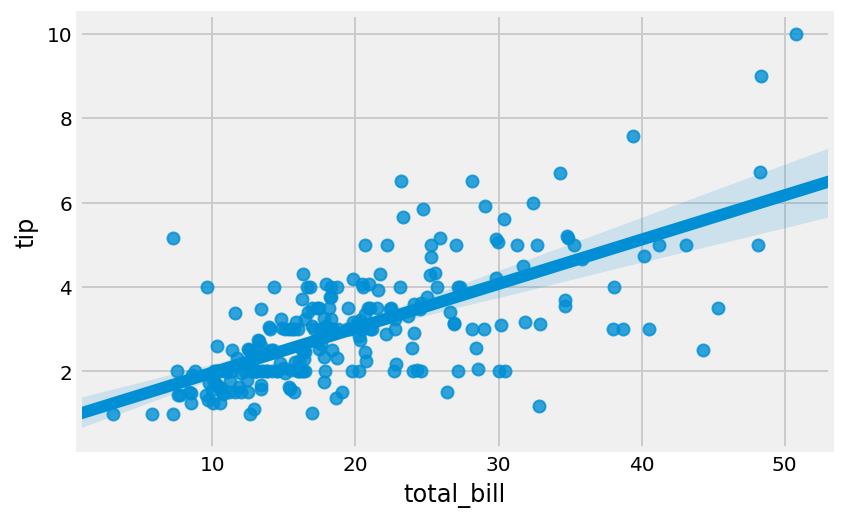5.  We can also visualize the distribution of categorical data with different types of plots. Here, we display a bar plot, a violin plot, and a swarmplot, which show an increasing amount of details:

```f, (ax1, ax2, ax3) = plt.subplots(
1, 3, figsize=(12, 4), sharey=True)
sns.barplot(x='sex', y='tip', data=tips, ax=ax1)
sns.violinplot(x='sex', y='tip', data=tips, ax=ax2)
sns.swarmplot(x='sex', y='tip', data=tips, ax=ax3)
```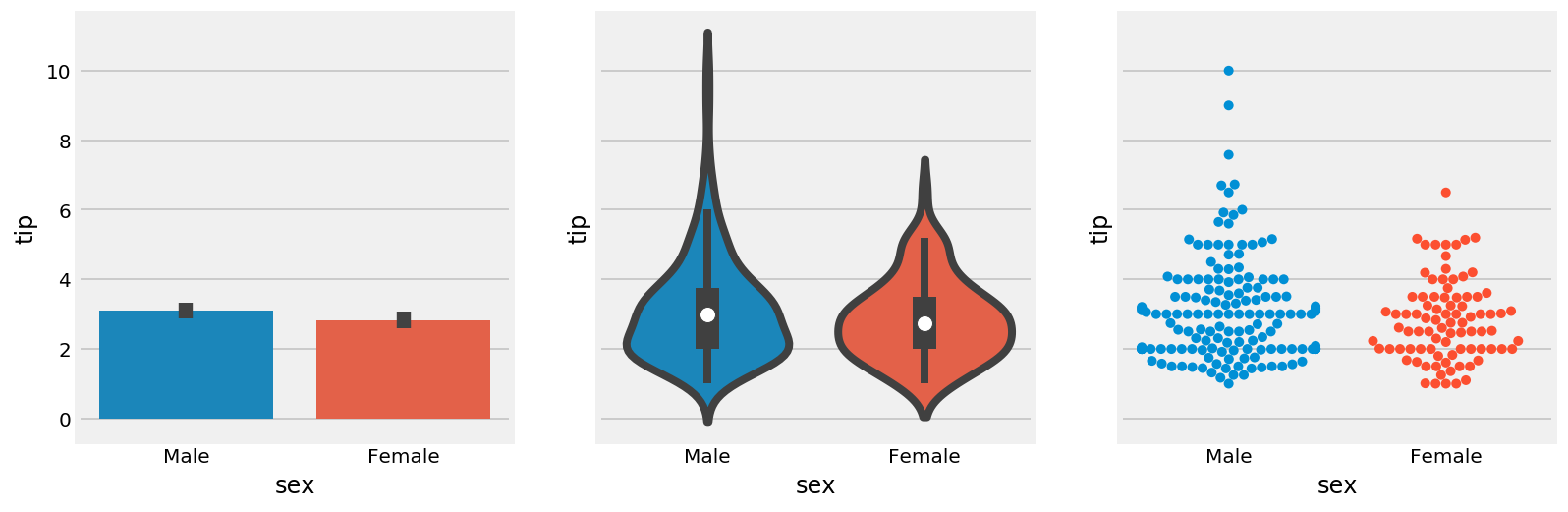The bar plot shows the mean and standard deviation of the tip, for males and females. The violin plot shows an estimation of the distribution in a more informative way than the bar plot, especially with non-Gaussian or multimodal distributions. The swarm plot displays all points, using the x axis to make them non-overlapping.

6.  The `FacetGrid` lets us explore a multidimensional dataset with several subplots organized within a grid. Here, we plot the tip as a function of the bill with a linear regression, for every combination of smoker (Yes/No) and sex (Male/Female):

```g = sns.FacetGrid(tips, col='smoker', row='sex')
g.map(sns.regplot, 'total_bill', 'tip')
```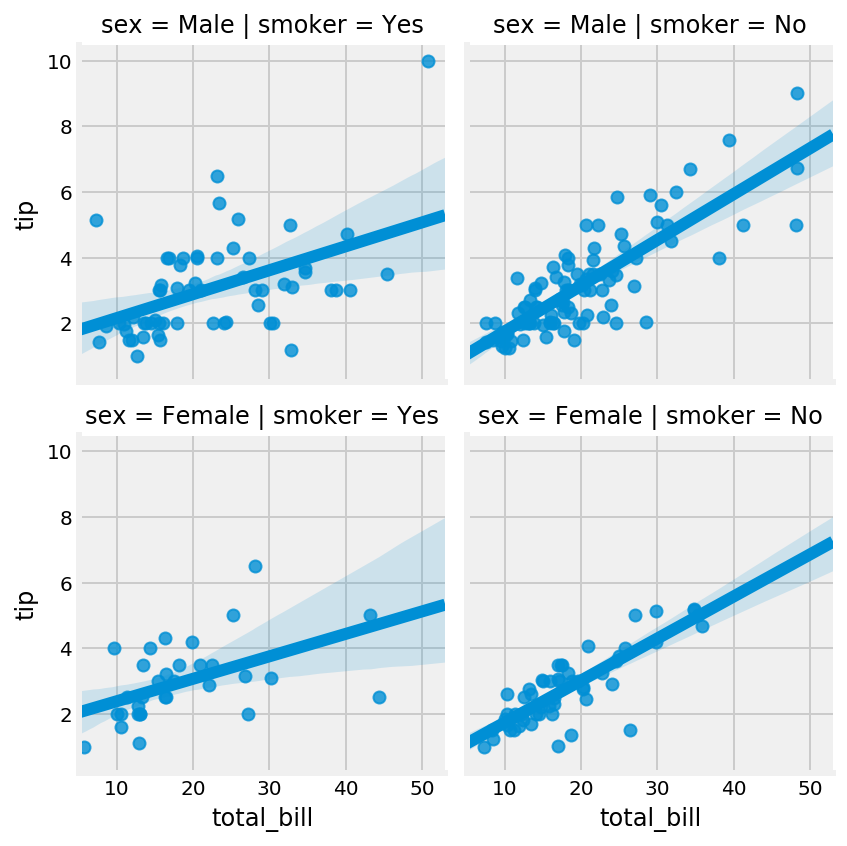## There's more...

Besides seaborn, there are other high-level plotting interfaces:

• The Grammar of Graphics is a book by Dr. Leland Wilkinson that has influenced many high-level plotting interfaces such as R's ggplot2, Python's ggplot by ŷhat, and others.
• Vega, by Trifacta, is a declarative visualization grammar that can be translated to D3.js (a JavaScript visualization library). Altair provides a Python API for the Vega-Lite specification (a higher-level specification that compiles to Vega).

Here are some more references: